# Generalized functions, product of

(diff) ← Older revision | Latest revision (diff) | Newer revision → (diff)

The product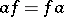of a generalized functionin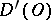and a function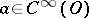is defined by the equation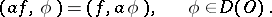Here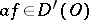, and for (ordinary) functionsin, the productcoincides with the ordinary product of the functionsand.

## Contents

### Examples.

1)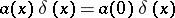;

2)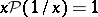.

However, this product operation cannot be extended to arbitrary generalized functions in such a way that it is associative and commutative, otherwise there would be the contradiction: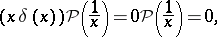In order to define the product of two generalized functionsand, it is sufficient for them to possess, roughly speaking, the following properties: "non-regularity" ofin a neighbourhood of any point must be compensated by corresponding "regularity" of, and conversely; for example, if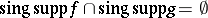(see Support of a generalized function). A product can be defined in certain classes of generalized functions, but it may turn out not to be uniquely determined.

### Examples.

3) The boundary values of the algebra of holomorphic functions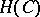(one-frequency generalized functions):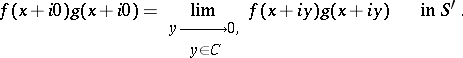They form an associative and commutative algebra with an identity .

4)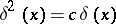, whereis an arbitrary constant. In fact,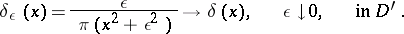But on test functionsfor which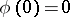,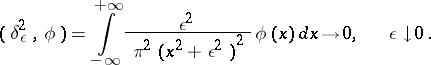Hence it is natural to put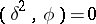if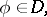. Extending this functional to all test functionsin, one obtains 4).

5) The definition of the product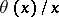. The functiondoes not belong to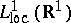, but it defines regular generalized functions:in,, and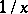in,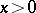. They can be consistently extended to generalized functions in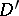, for example, by taking the finite Hadamard part of the divergent integral (renormalizing it)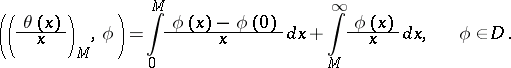The generalized function(the renormalized functional for) depends on the arbitrary parameter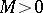. The arbitrariness in the renormalization is the following:These ideas lead to the procedure of renormalization of Feynman amplitudes in quantum field theory. The renormalization constants (for examples, masses and charges) appear as arbitrary constants, like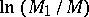; the most general definition of a product of generalized functions is given in terms of wave front sets.

How to Cite This Entry:
Generalized functions, product of. Encyclopedia of Mathematics. URL: http://encyclopediaofmath.org/index.php?title=Generalized_functions,_product_of&oldid=18083
This article was adapted from an original article by V.S. Vladimirov (originator), which appeared in Encyclopedia of Mathematics - ISBN 1402006098. See original article